# Silicon¶

This document is part of the QuantumWise Semiconductor Whitepapers. Here we investigate computational methods for ATK-DFT calculations for bulk silicon in the diamond crystal structure. We apply the SG15 pseudopotentials and a number of exchange-correlation functionals; PBE, PBEsol, TB09-MGGA, and PBE with the Pseudopotential Projector-Shift method. A limited number of HSE calculatios have been done for comparison. See the section Introduction for more information.

The following quantities have been calculated for each computational method, both at the experimental silicon lattice constant and at the theoretically predicted ones:

• Elastic constants: Bulk modulus, Poisson ratio, and Young’s modulus.
• Band structure along the X–Γ–L Brillouin zone path.
• Direct and indirect band gaps.
• Effective masses in the valence band maximum and conduction band minimum.
• Static dielectric constant.

Convergence studies indicate that a 9x9x9 k-point grid and a 100 Hartree density mesh cutoff yields a well-converged silicon lattice constant, and these settings were used for computing the silicon ground state electronic structure used in all the ATK-DFT analyses listed above.

The projector shifts used for the pps-PBE method are 11.23 eV for s-orbitals and -1.09 eV for p-orbitals. This yields a single computational method with fairly high accuracy for predicting the fundamental band gap and lattice constant for silicon. Note that these PPS parameters should only be used with SG15 pseudopotentials.

This document is organized as follows. The section Summary gives a short summary of the main results from this study, while the section Convergence briefly presents the convergence studies mentioned above, and the section Timing compares CPU timings and scalability of different SG15 basis sets for silicon. Next, the section Results contains figures illustrating the performance of the different computational methods in predicting the materials properties listed above. Finally, the Appendix gives a template QuantumATK Python script for setting up ATK-DFT calculations for silicon similar to the ones presented here, and also elaborates on how to calculate hole effective masses using ATK-DFT.

## Summary¶

The following list summarizes the main conclusions from this study.

• The PBEsol and pps-PBE methods both yield very accurate silicon lattice constants, but it is hard to say which method is most appropriate for computing elastic constants.
• All tested methods yield a qualitatively correct silicon band structure, although the position of the conduction band minimum depends a little on the method used.
• The TB09-MGGA, pps-PBE, and HSE methods accurately reproduce the fundamental (indirect) band gap of silicon.
• Electron effective masses in the silicon comduction band minimum are quite well captured by all tested methods. However, for hole effective masses in the valence band maximum, including spin-orbit interactions in the DFT calculations is crucial.
• As for the silicon static dielectric constant, the TB09-MGGA(PBE) and pps-PBE methods appear very accurate.

## Convergence¶

An optimum combination of Monkhorst–Pack k-point grid and density mesh cutoff energy must be chosen such as to maximize computational accuracy while minimizing the computational effort. The two figures below illustrate how the calculated silicon lattice constant depends on these two parameters.

We see from Fig. 195 that a 9x9x9 k-point grid is in general sufficient to get a well-converged silicon lattice constant: The convergence curves are similar across different combinations of exchange-correlation functionals and SG15 basis sets, and with a 9x9x9 grid and denser, the lattice constant is converged to within roughly 10-3 % of the most dense k-point grid tested (19x19x19).

Similarly, Fig. 196 shows that a density mesh cutoff energy of 100 Hartree is in general sufficient: The calculated silicon lattice constant is converged to within roughly 10-3 % of the most dense density mesh tested (200 Ha cutoff energy).

Note

All remaining ATK-DFT calculations in this study of pure silicon therefore use a 100 Hartree mesh cutoff energy and a 9x9x9 Monkhorst–Pack k-point grid.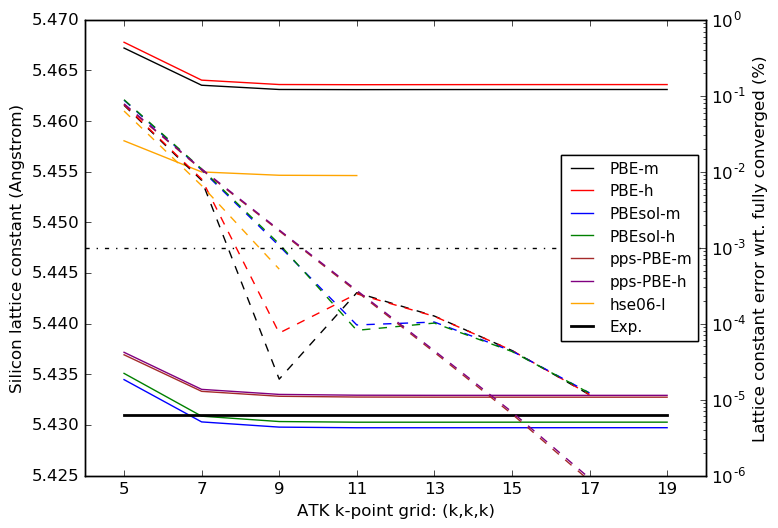Fig. 195 Convergence of the silicon lattice constant with respect to k-point sampling (using Monkhorst-Pack grids). For the QuantumATK calculations the PBE and PBEsol exchange-correlation functionals, and PBE with the pseudopotential projector-shift (pps-PBE) have been used. Both the medium (m) and high (h) SG15 basis sets with 200 Hartree mesh cutoff energy have been used. HSE calculations have been performed using FHI-AIMS and the light basis set. The dashed lines indicate the (absolute) relative difference to the fully converged lattice constant (right-hand y-axis, in percent).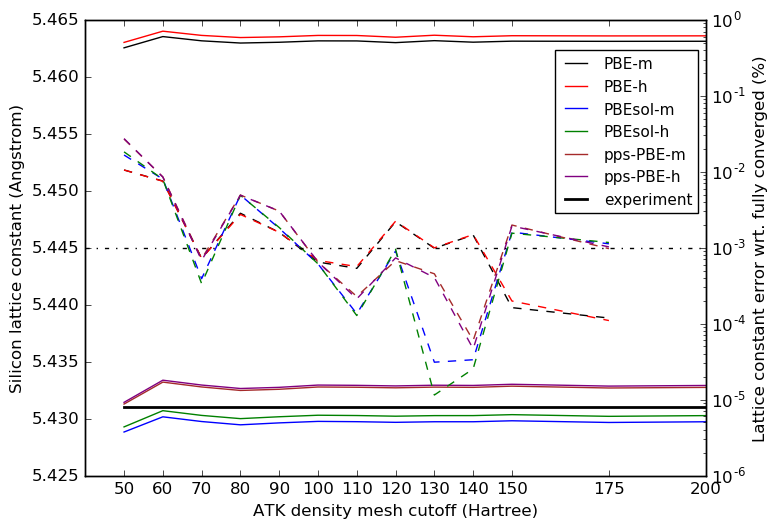Fig. 196 Convergence of the silicon lattice constant with respect to the QuantumATK mesh cutoff energy using the PBE and PBEsol exchange-correlation functionals, and PBE with the pseudopotential projector-shift (pps-PBE), all for both the medium (m) and high (h) SG15 basis sets with a 15x15x15 k-point grid. The dashed lines indicate the (absolute) relative difference to the fully converged lattice constant (right-hand y-axis, in percent).

## Timing¶

The computational cost of a calculation may depend critically on the choice of basis set, particularly for calculations on large systems with many atoms. Fig. 197 shown below illustrates this for the present case of silicon and the Medium, High, and Ultra SG15 basis sets.

The silicon primitive cell (containing 2 atoms) was systematically repeated in steps of one along all three lattice vectors, resulting in rapidly increasing systems sizes. PBE calculations were then performed for each system, using Γ-point sampling only, to avoid any influence of k-point sampling on the recorded CPU times.

It is quite clear from the figure that the computational loads of the SG15 Medium and High basis sets for silicon are not much different for relatively small system sizes, but the High basis set becomes significantly more demanding for larger system sizes. For the 432-atom silicon unit cell, the CPU time difference is roughly a factor of 2. Moreover, the Ultra basis set appears in comparison extremely demanding, and will not be used in the remaining calculations in this study.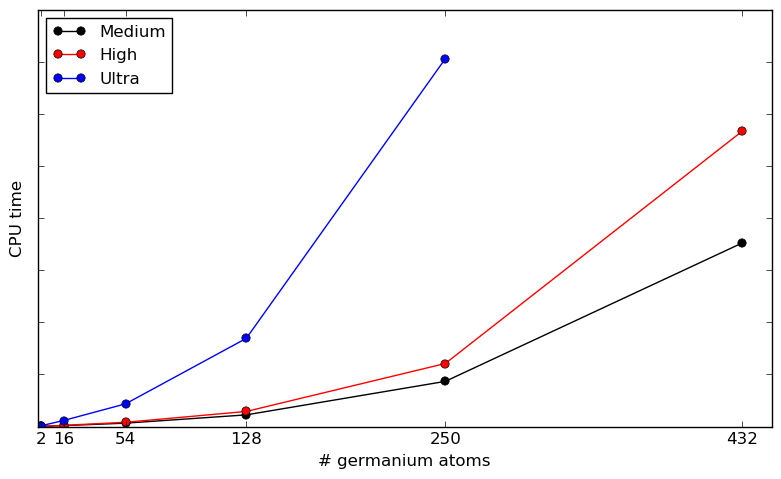Fig. 197 Total CPU time for the first 5 SCF iterations by the ATK-DFT calculator for the Medium (black), High (red), and Ultra (blue) SG15 basis sets, against the number of silicon atoms in the increasingly larger (repeated) silicon unit cell. Only the Γ point is sampled (1x1x1 k-point grid) and the calculations were executed on a single CPU. Note that both figure axes are linear.

## Results¶

This section presents results obtained with the PBE, PBEsol, pps-PBE, and TB09-MGGA methods using Medium and High basis sets with the SG15 pseudopotential for silicon. The list below gives direct links to all subsections:

### Lattice constant¶

The PBEsol lattice constant for silicon is very close to the experimental value, 5.431 Å, but the pps-PBE method is also very accurate. The bare PBE functional (without any pseudopotential projector shifts) is known to overestimate lattice constants in general, but for silicon only by 0.6%.Fig. 198 Silicon lattice constant calculated using QuantumATK and the PBE (blue), PBEsol (red) exchange-correlation functionals and the pps-PBE method (green), plotted as deviation from the experimental value. Both medium (m) and high (h) SG15 basis sets are used. HSE calculations have been performed using FHI-AIMS with light (l) and tight (t) basis sets.

### Elastic constants¶

Almost all methods tested appear to underestimate the silicon bulk modulus and Young’s modulus, but overestimate the Poisson ratio. The PBE-m method is a curious exception to this rule, but the pps-PBE method appears in general better.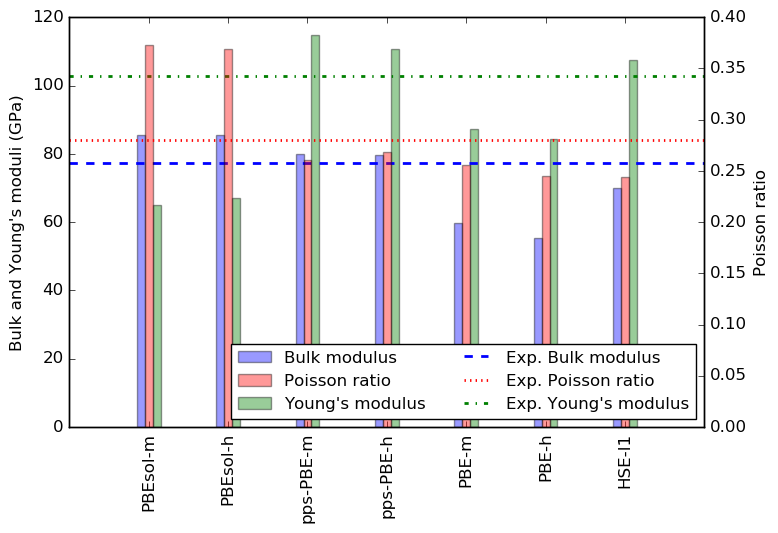Fig. 199 Silicon bulk modulus (blue), Poisson ratio (red), and Young’s modulus (green) calculated using the PBE, PBEsol, pps-PBE, and HSE methods. The horizontal lines indicate experimental values, all from www.crystran.co.uk.

### Band structure¶

All tested methods correctly predict a valence band maximum (VBM) at the Brillouin zone Γ point, and a conduction band minimum (CBM) close to the X point, along the Γ–X path. However, the position of the CBM depends slightly on the method applied, and the band energy in the CBM significantly so (see also section Band gaps). On the other hand, the Medium and High basis sets appear to give very similar silicon band structures.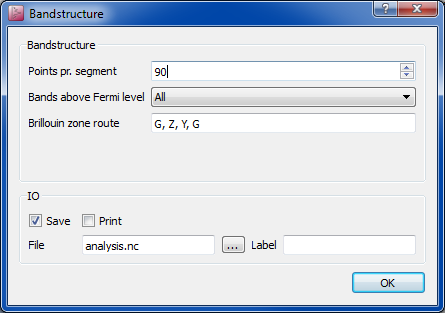Fig. 200 (a) Bandstructure of silicon calculated using QuantumATK with the PBE, pbesol and TB09-MGGA exchange-correlation functionals and with the pps-PBE method. The bandstructure has also been calculated using FHI-AIMS and the HSE functional. All the plotted band structures are aligned at the valence band maximum (VBM). Both medium (m) and high (h) SG15 basis sets are used in the QuantumATK calculations. In the FHI-AIMS calcualtions, light (l) and tight (t) basis sets have been used. The name in parenthesis in the labels refers to the lattice constant: ‘exp’ means lattice constant fixed at the experimental value, 5.431 Angstrom, while ‘PBE, ‘PBEsol’, and ‘pps-PBE’ indicate that the lattice constant was optimized using DFT. The shaded black area indicates the region around the conduction band minimum (CBM) shown in panel b. (b) Zoom around the CBM of the data shown in panel a. The dashed black line marks the position of the calculated CBMs.

### Band gaps¶

The silicon fundamental band gap is well reproduced by the TB09-MGGA, pps-PBE, and HSE methods, both at experimental and DFT optimized lattice constants, the pps-PBE yielding a particularly accurate band gap. Note, however, that the TB09-MGGA c-parameter was calculated self-consistently from the silicon electronic structure (no fitting), while the pps-PBE projector shifts were essentially fitted to the silicon band gap and lattice constant. On the other hand, the TB09-MGGA functional cannot be used for geometry optimization, which the pps-PBE method is well suited for. Also note that the HSE direct gap, $$E_{\Gamma_1}$$, is very accurate.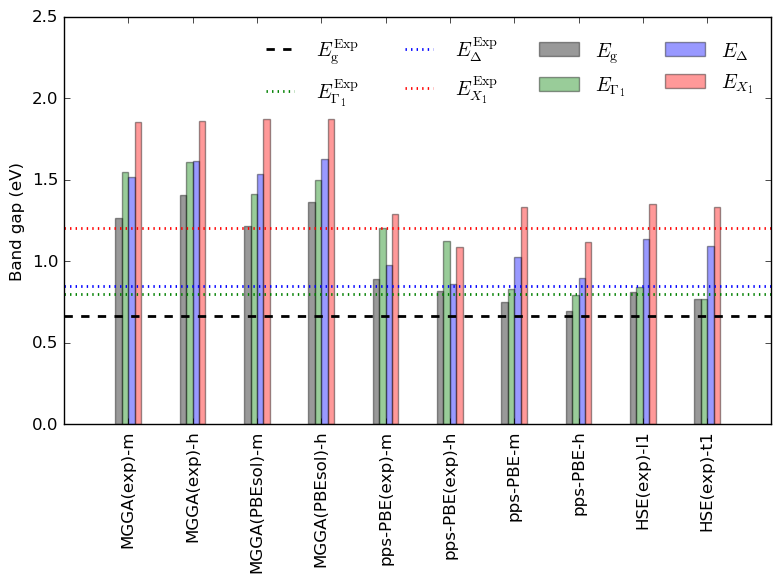Fig. 201 Silicon band gaps; $$E_\mathrm{g}$$ (fundamental gap, black) and energy gaps from the $$\Gamma$$-point valence band maximum to the conduction band minima at X (red), L (blue), and $$\Gamma$$ (green), calculated using the PBE, PBEsol, and TB09-MGGA, HSE (using FHI-AIMS) exchange-correlation functionals and with the pps-PBE method. Both medium (m) and high (h) SG15 basis sets are used, while for the HSE calculations the FHI-AIMS light and tight are used. The name in parenthesis in the labels on the x-axis refers to the lattice constant: ‘exp’ means lattice constant fixed at the experimental value, 5.431 Angstrom, while ‘pbe’, ‘pbesol’, ‘pps-pbe’ and ‘hse’ indicate that the lattice constant was optimized using DFT and the corresponding functional. The reference values $$E_\mathrm{g}^\mathrm{Exp}$$ (black, dashed), $$E_{X_1}^\mathrm{Exp}$$ (red, dotted), $$E_{L_1}^\mathrm{Exp}$$ (blue, dash-dotted), and $$E_{\Gamma_1}^\mathrm{Exp}$$ (green, dotted) are from http://www.ioffe.ru/SVA/NSM/Semicond/Si/Figs/121.gif.

### Effective masses¶

The silicon effective masses that are calculated with spin-orbit interaction included are in fair agreement with experiment for all the density functionals, basis sets, and pseudopotentials adopted in this study, see the two figures and table in this section. There exists a certain trend that the hole effective mass values tend to be somewhat underestimated as compared to experimental values for the LDA and GGA-PBE density functionls, which underestimate the silicon band gap, whereas the hole effective masses for the TB09-MGGA and pps-PBE density functionals, which give accurate band gap for bulk Si, tend to be overestimated. The electron longitudinal and transversal masses calculated at the X-valley minimum are relatively insensitive to the choice of density functional. Having the spin-orbit interaction included into the effective mass calculation is crucial for reproducing the experimental effective mass values.Fig. 202 Silicon effective masses calculated with different methods, basis sets, and lattice parameters, without including spin-orbit interaction. The LDA effective masses calculated with the FHI pseudopotential and DoubleZetaPolarized basis set are given with red dashed and red dotted lines for the longitudinal and transversal electron masses, respectively. The yellow, green, and blue lines stand for the heavy, light, and split-off hole effective masses for different crystollagraphic directions: the (100) direction (dotted line), (111) direction (dashed line), and (110) direction (dot-dashed line). The LDA results are taken as a reference for the case of no spin-orbit coupling, since the LDA derived effective masses of Si calculated with spin-orbit coupling are in good agreement with experiment. Note that the same color scheme is chosen for the ATK-DFT calculated effective mass data that are given with color bars.Fig. 203 Silicon effective masses calculated with different methods, basis sets, calculations. The experimental heavy, light, and split-off effective masses are averaged over the three crystallographic directions, and are given with yellow dashed, green dotted, and blue dot-dashed line, respectively. The red dashed and dotted lines correspond to the longitudinal and transversal electron masses measured in experiment, respectively. Note that the same color scheme is chosen for the ATK-DFT calculated effective mass data that are given with color bars. For the sake of comparison, the LDA-ABINIT results for effective masses obtained by Janssen et al. [Phys. Rev. B 93, 205147 (2016)] with and without spin-orbit interaction included are added to the figure together the experimental data for different crystallographic directions, see R. N. Dexter and B. Lax, Phys. Rev. 96, 223 (1954). The experimental light-hole effective mass is from http://www.ioffe.ru/SVA/NSM/Semicond/Si/bandstr.html.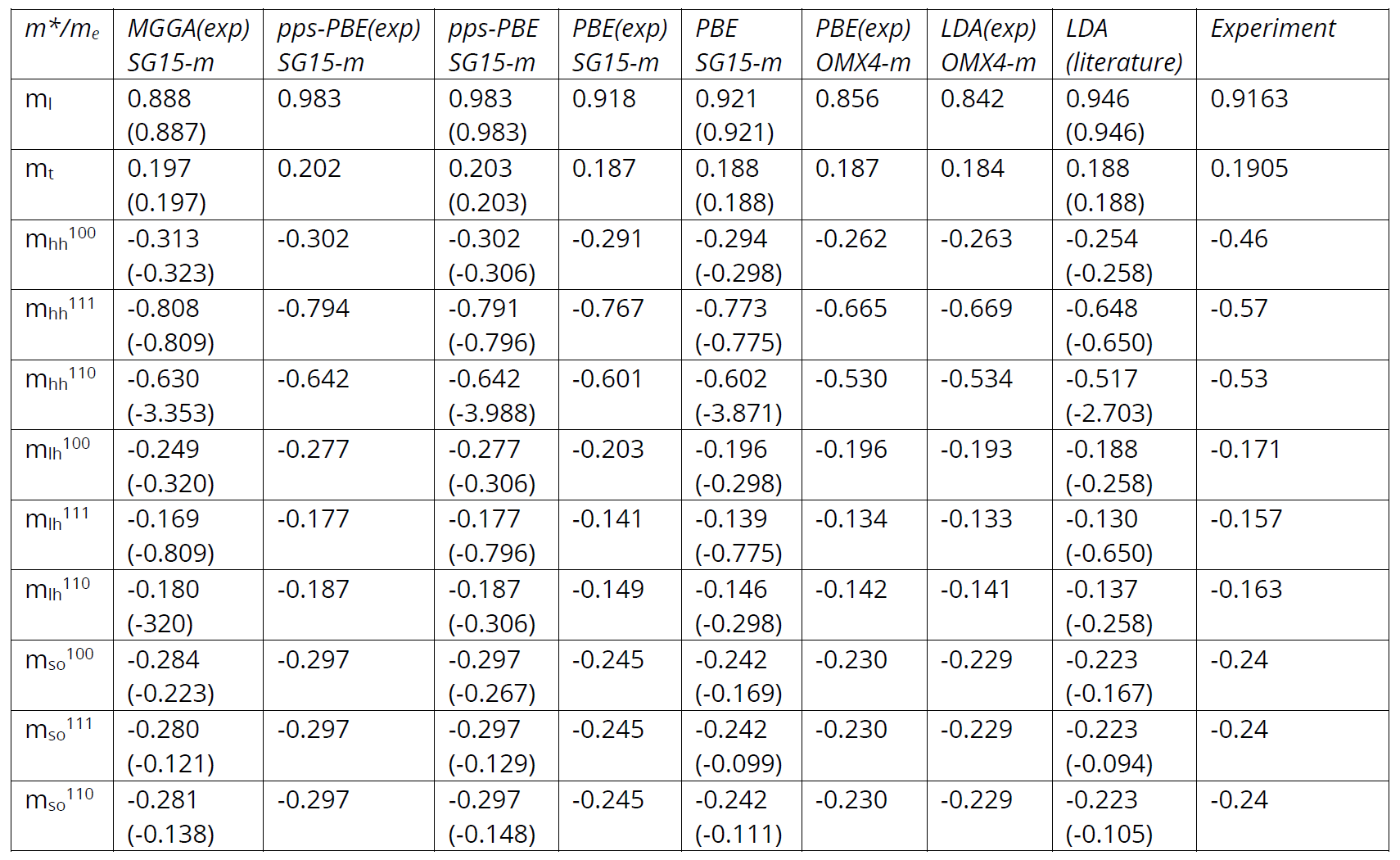Fig. 204 Table of silicon effective masses calculated with different computational methods, all including spin-orbit interaction. The literature LDA+SO data were calculated using the ABINIT plane-wave code [JGP+16], while the experimental data for the heavy and light hole effective masses are from [DZL56] and from http://www.ioffe.ru/SVA/NSM/Semicond/Si/bandstr.html for split-off masses. For the sake of comparison, effective masses calculated without including spin-orbit interaction are provided in parenthesis.

### Dielectric constant¶

The static ($$\omega=0$$) dielectric constant of the silicon bulk is most reliably reproduced by the TB09-MGGA functional at the PBE lattice constant and by the pps-PBE method at the corresponding theoretical lattice constant. The lattice constant used seems in general to significantly affect the calculated dielectric constant; note for example how the PBE dielectric constant changes from 11 to 14 depending on the lattice constant used, but independently of the basis set.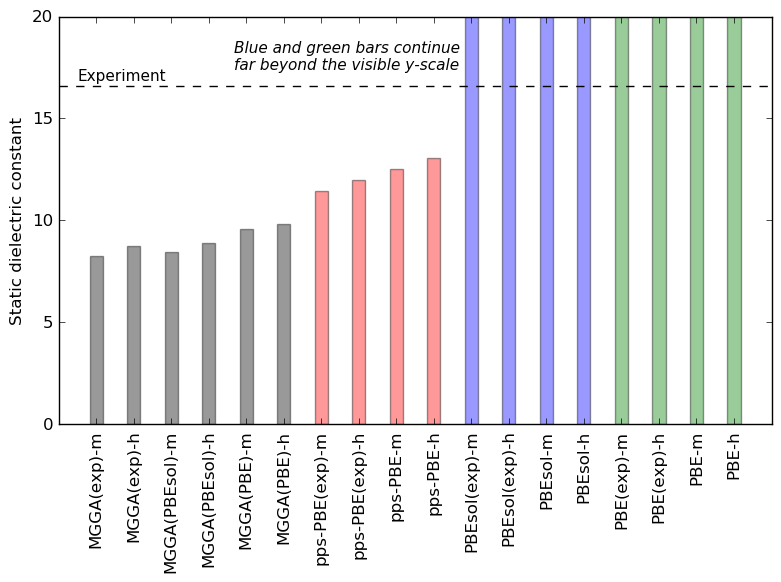Fig. 205 Static dielectric constant calculated using the TB09-MGGA (black), PBE (red) and PBEsol (blue) exchange-correlation functionals and the pps-PBE method (green). Both medium (m) and high (h) SG15 basis sets are used, and the number of included electronic bands were 10 below the Fermi level and 20 above it. The name in parenthesis in the labels on the x-axis refers to the lattice constant: ‘exp’ means lattice constant fixed at the experimental value, 5.431 Angstrom, while ‘PBE, ‘PBEsol’, and ‘pps-PBE’ indicate that the lattice constant was optimized using DFT. The dashed line indicates the exprimental value, 11.9.

## Appendix¶

The QuantumATK Python script shown below may be used as a template for ATK-DFT calculations for silicon with the SG15 pseudopotential. The script defines the silicon bulk configuration and then sets up the ATK-DFT calculator with PBE exchange-correlation. The script blocked named Basis Set shows various options for the SG15 basis set; ordinary PBE and PBE with pseudopotential projector shifts, both with Medium and High basis sets.

The script is available for direct download: silicon.py.

  1 2 3 4 5 6 7 8 9 10 11 12 13 14 15 16 17 18 19 20 21 22 23 24 25 26 27 28 29 30 31 32 33 34 35 36 37 38 39 40 41 42 43 44 45 46 47 48 49 50 51 52 53 54 55 56 57 58 59 60 61 62 63 64 65 66 67 # ------------------------------------------------------------- # Bulk Configuration # ------------------------------------------------------------- # Set up lattice lattice = FaceCenteredCubic(5.431*Angstrom) # Define elements elements = [Silicon, Silicon] # Define coordinates fractional_coordinates = [[ 0. , 0. , 0. ], [ 0.25, 0.25, 0.25]] # Set up configuration bulk_configuration = BulkConfiguration( bravais_lattice=lattice, elements=elements, fractional_coordinates=fractional_coordinates ) # ------------------------------------------------------------- # Calculator # ------------------------------------------------------------- #---------------------------------------- # Basis Set #---------------------------------------- # Ordinary GGA: Medium or High basis set for SG15. basis_set = [BasisGGASG15.Silicon_Medium] #basis_set = [BasisGGASG15.Silicon_High] # GGA with pseudopotential projector-shift method: Medium or High basis set for SG15. #projector_shift = PseudoPotentialProjectorShift(s_orbital_shift=11.23*eV, # p_orbital_shift=-1.09*eV) #basis_set = [BasisGGASG15.Silicon_Medium(projector_shift=projector_shift)] #basis_set = [BasisGGASG15.Silicon_High(projector_shift=projector_shift)] #---------------------------------------- # Exchange-Correlation #---------------------------------------- exchange_correlation = GGA.PBE k_point_sampling = MonkhorstPackGrid( na=9, nb=9, nc=9, ) numerical_accuracy_parameters = NumericalAccuracyParameters( k_point_sampling=k_point_sampling, density_mesh_cutoff=100.0*Hartree, ) iteration_control_parameters = IterationControlParameters( damping_factor=0.4, ) calculator = LCAOCalculator( basis_set=basis_set, exchange_correlation=exchange_correlation, numerical_accuracy_parameters=numerical_accuracy_parameters, iteration_control_parameters=iteration_control_parameters, ) bulk_configuration.setCalculator(calculator) nlprint(bulk_configuration) bulk_configuration.update() nlsave('silicon.nc', bulk_configuration) 

### Calculation of effective masses¶

The following is a list of important points abouth calculations of masses using ATK-DFT. See also section Effective masses.

1. As seen from Fig. 202, the SG15_medium basis set is rather accurate for calculating the electron and hole effective masses of Si, as suggested by comparison of effective masses obtained with the SG15 Medium and SG15 High basis sets.
2. The calculated effective masses are not sensitive to the choice of either DFT-derived or experimental lattice parameter value for DFT calculations, see Fig. 202 and Fig. 203, i.e., the electron and hole effective masses of Si depend weakly on the lattice constant near its experimental value.
3. The ATK-DFT-LCAO calculations of effective masses are in consistency with the corresponding plane-wave pseudopotential calculations done with the ABINIT code, see Janssen et al. [Phys. Rev. B 93, 205147 (2016)], which provides a reference for the quality of the LCAO basis set. The agreement does not depend on whether the calculations are done with or without spin-orbit interaction included.
4. In general, the spin-orbit interaction has to be taken into account for hole effective mass calculations to achieve a good agreement with experiment. Otherwise, the effective masses are unphysically anisotropic and are heavily overestimated as seen in the first figure and the table. Unlike the hole effective masses, the longitudinal and transversal electron effective masses are both virtually unaffected by the spin-orbit interaction.
5. The electron, light, and split-off hole effective masses calculated using the LDA and GGA-PBE functionals with the SG15 basis set are closer to the corresponding experimental values, compared to the masses obtained with the OMX basis set. The heavy hole masses seem to be in a better agreement with experiment if obtained with the OMX basis set. Note that the LDA and GGA functional underestimate the indirect band gap of Si by roughly a factor of 2, as compared to experiment.
6. Using the TB09 meta-GGA and pps-GGA-PBE functionals corrects for the band gap value mentioned in #5. At the same time, it results in hole effective mass values that are larger than the LDA and GGA-PBE derived masses, and are somewhat overestimated compared to the corresponding experimental values. That might be explained by the fact that the TB09 meta-GGA and pps-GGA-PBE calculated band gap increases as compared to the LDA and GGA-PBE functionals, so that it drives the system into more insulating state. That is consistent with the increase of the hole effective masses. We also notice that using the pps-GGA-PBE functional, which has adjustable parameters, allows correcting the lattice constant value of Si (in addition to correcting the band gap) that is otherwise highly overestimated compared to the experimental value if a standard GGA-PBE functional is adopted for volume optimization of the Si unit cell.
1. There exists a subtle issue in hole effective mass calculation for degenerate bands at the G point. The accurate extraction of effective masses with a finite-difference method requires calculating the effective mass for a given crystallographic direction as a function of the k-mesh density by varying ‘delta’ parameter (which is a k-mesh descretization step) in the EffectiveMass analyzer. The actual effective mass value is then to be taken in a parabolic band region where the corresponding mass values should show virtually no ‘delta’ dependence, i.e., the effective mass as a function of ‘delta’ (k-mesh density) reaches a plateau. Note that the default value of 0.001 Å-1 for ‘delta’ is good enough for calculating electron and split-off masses, but it may need to be increased to ~0.01 Å-1 for light and heavy hole masses. The TB09-MGGA derived light and heavy hole effective masses were calculated at even higher value of delta ~0.03 Å-1.